# Basics of Logic Practice 03

## Counting Figures Practice

Let’s do counting figures practice to learn how to analyze a different situation in a bit tricky way. These type of question may come in the quantitative section, but it’s basics of analytical in nature.

1. If four lines are drawn on a circle, in maximum how many parts will those lines divide that circle?

A) 6
B) 8
C) 9
D) 11
E) 12

2. How many triangles can you spot in the figure below?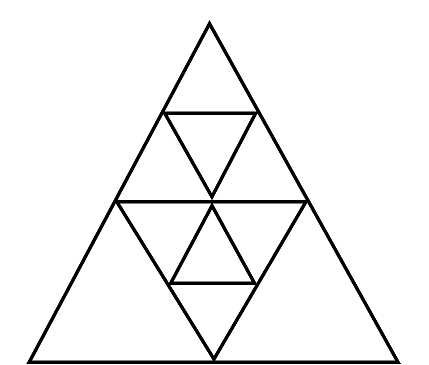A) 5
B) 7
C) 9
D) 11
E) 13

3. How many triangles are there in the bellow figure?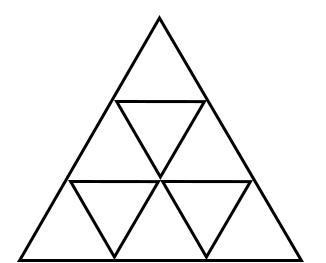A) 5
B) 7
C) 9
D) 11
E) 13

4. How many squares exist in the following figure?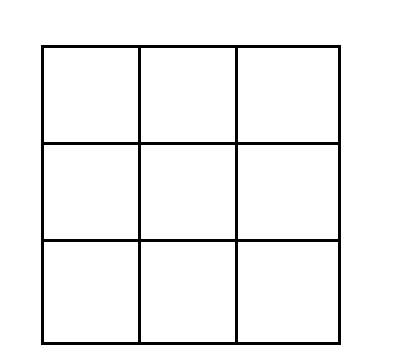A) 5
B) 7
C) 9
D) 11
E) 13

5. Find all the possible triangles that you can see in below figure: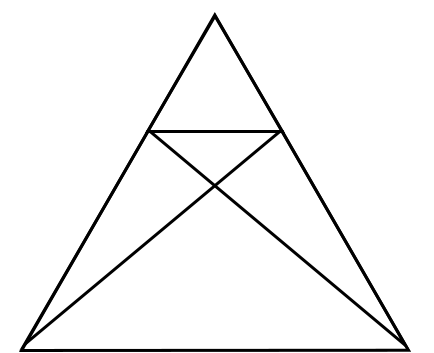A) 5
B) 7
C) 9
D) 11
E) 13

6. How many rectangles can you see in the diagram below?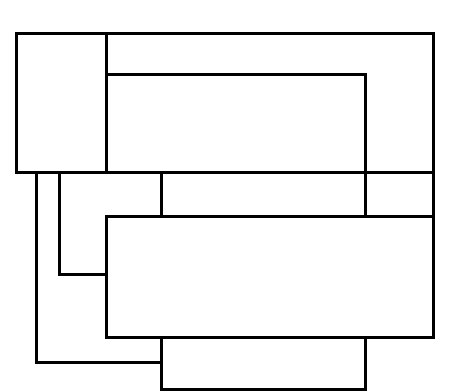A) 5
B) 7
C) 9
D) 11
E) 13

7. How many triangles are there inside the square below?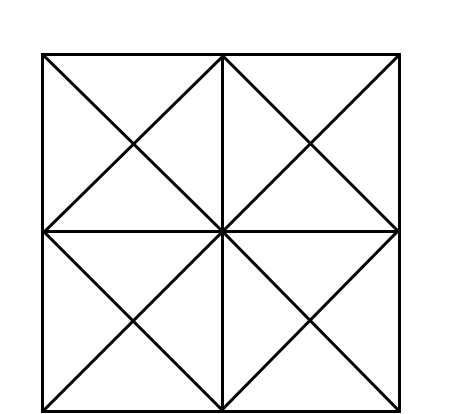A) 5
B) 7
C) 9
D) 11
E) 13

8. Can you find the correct number of possible triangles in the below diagram?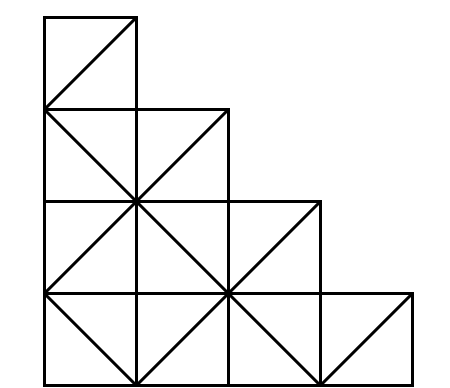A) 5
B) 7
C) 9
D) 11
E) 13

9. In the figure below, the sum of all possible rectangles and triangles equals what number? (Note: Square is also a type of rectangle)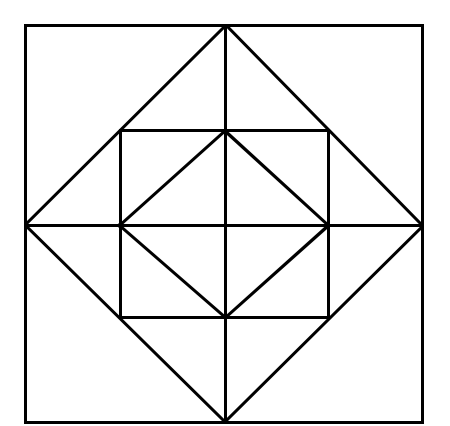A) 5
B) 7
C) 9
D) 11
E) 13

10. How many possible triangles can you spot in the figure below?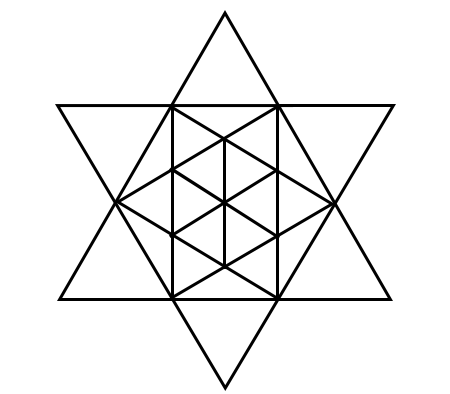A) 5
B) 7
C) 9
D) 11
E) 13

Don’t go ahead for the solution before trying it by yourself. Give at least 3 – 5 minutes on every question for brainstorming to get your version and see if it is correct!